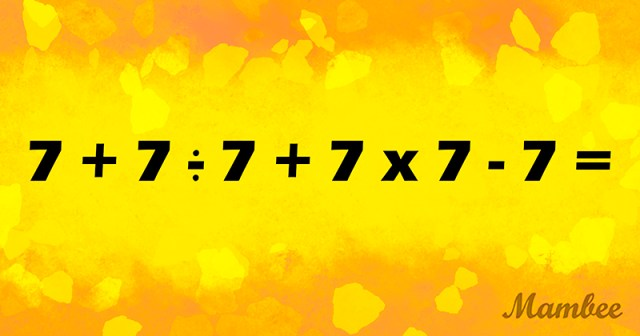# A Brain Workout: Can You Solve This Viral Math Equation On The First Try?

At first glance, equations like these appear to be straightforward. However, before you begin, a word of caution: if you do not follow the correct equation sequence, you may not be able to complete it. This issue has been discussed millions of times on the Internet and has perplexed many individuals.

So, are you prepared to carry out the proper procedure in the correct order?

Scroll down to get the answer:

.

.

.

.

.

We multiply and divide since we don’t have any parenthesis or exponents.

To begin, we divide 7 by 7, yielding 1. After that, we tackle multiplication: 7 x 7 = 49.

You’re doing a fantastic job!

The next step is to plug these numbers into the following equation:

Now we have 7 + 1 + 49 – 7 = ?

It means that there are only the addition and subtraction left. Let’s do it from left to right:

7+1 = 8

8+49 = 57

And finally, 57 – 7 = 50!